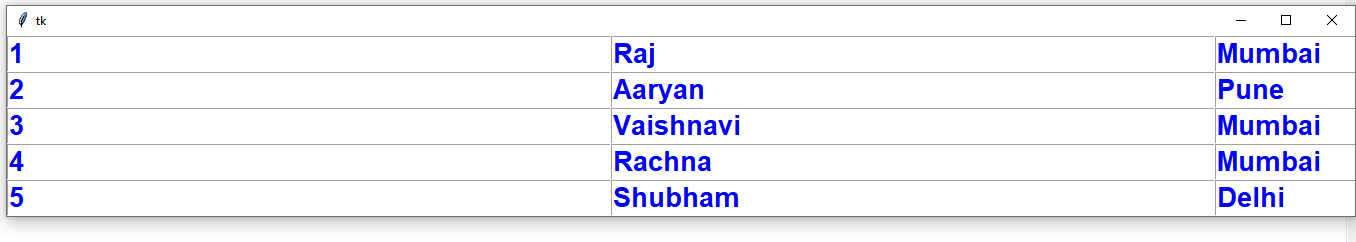# Create Table Using Tkinter

## Create Table Using Tkinter

Python offers multiple options for developing a GUI (Graphical User Interface). Out of all the GUI methods, Tkinter is the most commonly used method. It is a standard Python interface to the Tk GUI toolkit shipped with Python. Python with Tkinter is the fastest and easiest way to create GUI applications. Creating a GUI using Tkinter is an easy task.

## Creating Tables Using Tkinter

A table is useful to display data in the form of rows and columns. Unfortunately, Tkinter does not provide a Table widget to create a table. But we can create a table using alternate methods. For example, we can make a table by repeatedly displaying entry widgets in the form of rows and columns.

To create a table with five rows and four columns we can use two for loops as:

```for i in range(5):
for j in range(4):
```

Inside these loops, we have to create an Entry widget by creating an object of Entry class, as:

```e = Entry(root, width=20, fg='blue', font=('Arial', 16, 'bold')
```

Now, we need logic to place this Entry widget in rows and columns. This can be done by using `grid()` method to which we can pass row and column positions, as:

```# here i and j indicate
# row and column positions
e.grid(row=i, column=j)
```

We can insert data into the Entry widget using `insert()` method, as:

```e.insert(END, data)
```

Here, ‘END’ indicates that the data continuous to append at the end of previous data in the Entry widget.

This is the logic that is used in the program given below using the data that is coming from a list. We have taken a list containing 5 tuples and each tuple contains four values which indicate student id, name, city and age.

Hence, we will have a table with 5 rows and 4 columns in each row. This program can also be applied on data coming from a database to display the entire data in the form of a table.

Source Code:

 `# Python program to create a table` `  ` `from` `tkinter ``import` `*` ` ` ` ` `class` `Table:` `     ` `    ``def` `__init__(``self``,root):` `         ` `        ``# code for creating table` `        ``for` `i ``in` `range``(total_rows):` `            ``for` `j ``in` `range``(total_columns):` `                 ` `                ``self``.e ``=` `Entry(root, width``=``20``, fg``=``'blue'``,` `                               ``font``=``(``'Arial'``,``16``,``'bold'``))` `                 ` `                ``self``.e.grid(row``=``i, column``=``j)` `                ``self``.e.insert(END, lst[i][j])` ` ` `# take the data` `lst ``=` `[(``1``,``'Raj'``,``'Mumbai'``,``19``),` `       ``(``2``,``'Aaryan'``,``'Pune'``,``18``),` `       ``(``3``,``'Vaishnavi'``,``'Mumbai'``,``20``),` `       ``(``4``,``'Rachna'``,``'Mumbai'``,``21``),` `       ``(``5``,``'Shubham'``,``'Delhi'``,``21``)]` `  ` `# find total number of rows and` `# columns in list` `total_rows ``=` `len``(lst)` `total_columns ``=` `len``(lst[``0``])` `  ` `# create root window` `root ``=` `Tk()` `t ``=` `Table(root)` `root.mainloop()`

Output:Last Updated on November 13, 2021 by admin

## chr() in Pythonchr() in Python

chr() in Python The chr() method returns a string representing a character whose Unicode code point is

## Python infinityPython infinity

Python infinity As ironic as it may seem infinity is defined as an undefined number

Python OpenCV | cv2.imread() method OpenCV-Python is a library of Python bindings designed to solve computer

## Difference of two columns in Pandas dataframeDifference of two columns in Pandas dataframe

Difference of two columns in Pandas dataframe   Difference of two columns in pandas dataframe

## Permutation and Combination in PythonPermutation and Combination in Python

Permutation and Combination in Python Python provides direct methods to find permutations and combinations of

## Generating Random id’s using UUID in PythonGenerating Random id’s using UUID in Python

Generating Random id’s using UUID in Python In this article we would be using inbuilt

## Pandas DataFrame.isinPandas DataFrame.isin

When working with Pandas DataFrames, there are often cases where we need to check if

## Python – Pandas dataframe.mean()Python – Pandas dataframe.mean()

Python – Pandas dataframe.mean()   Pandas dataframe.mean() function return the mean of the values for